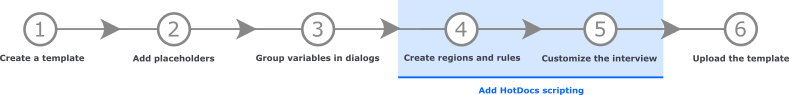# DATE OF Function

The DATE OF function returns a day, month, and year date value by formatting the three number values you specify as inputs to the function.

You can use this function when you need to calculate a date where one or more parts of the date (day, month, or year) are based on other answers. Replace day:NUM, month:NUM, and year:NUM placeholders with appropriate number values, which can come from Number variables, computations that return number values, or literal number values. For example, if your template needs a date value for New Year's Day in the current year, the day and month are both fixed (1), but the year is not known until the interview is asked or the document is assembled.

 Function name DATE OF Usage model DATE OF ( day:NUM, month:NUM year:NUM ) Parameters This function requires you to replace two of three parameters: day: NUM The function assembles a date value based on the other two values. month: NUM The function assembles a date value based on the other two values. year: NUM The function assembles a date value based on the other two values. Result A date value.

## Example

An employment contract should include language about an annual bonus, but only if the hire date is before August 15th of the current year. The following expression returns a date value for that date, where literal number values are used for the day (15) and month (8), and a number value computed using the YEAR OF function is used for the year:

```DATE OF ( 15, 8, YEAR OF( TODAY ) )
```

You can then use that expression in an IF instruction to compare it with the employee's hire date and create an appropriate message in each case:

```IF Hire Date <= DATE OF ( 15, 8, YEAR OF( TODAY ) )
Employee Name + " qualifies for the annual bonus."
ELSE
Employee Name + " does not qualify for this year's annual bonus."
END IF
```